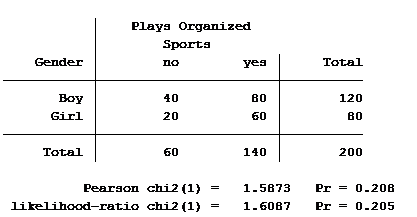# Understanding Incidence Rate Ratios through the Eyes of a Two-Way Table

by

by Jeff Meyer

The coefficients of count model regression tables are shown in either logged form or as incidence rate ratios. Trying to explain the coefficients in logged form can be a difficult process.

Incidence rate ratios are much easier to explain. You probably didn’t realize you’ve seen incidence rate ratios before, expressed differently.

Let’s look at an example.

A school district was interested in how many children in their sixth grade classes played on organized sports teams. So they did a count and also noted the gender of the child. The results were put into a table:

 Plays Organized Sports Gender No Yes Total Boy 40 80 120 Girl 20 60 80 Total 60 140 200

We can see from the table that the ratio of the number of girls to boys in the sixth grade classes is 80 to 120 or 0.667 to 1.

The ratio of the number of those who play sports to the number who do not is 140 to 60 or 2.333 to 1. (We learned about ratios back when we were in sixth grade.)

Girls:Boys = 80/120 = .667

Play Sports:Not Play Sports = 140/60 = 2.333

We don’t usually focus on these ratios in a 2×2 table. Instead, the focus is on the proportions.

Proportion of Girls = 80/200 = .40

Proportion of Boys = 120/200 = .60

What’s interesting, though, is the ratio of girls:boys is the same as the ratio of their proportions:

.40:.60 = .667

The school district superintendent decided to get fancy and create a statistical model. Because we counted the number of boys and girls who played or didn’t play sports, the superintendent suggested we use a count model.

The frequency for each of the four groups (gender by played sports) was the count model’s outcome variable. Our two predictor variables were gender and whether the child played sports.

Not knowing which type of count model to run, the superintendent decided to use a Poisson model. Here are the results:Here is the statistical software generated two-way table between gender and played sports:What do you notice from the results?

The incidence rate ratio (IRR) for girl’s is 0.6667. This is the same as the ratio of girls to boys in the table.

The incidence rate ratio for those who played sports to those who did not is 2.3333. This is also the same as the ratio of the number who played sports to the number who did not.

What else do we find?

The Pearson value divided by the degrees of freedom is 1.587, identical to the Pearson chi-square value in the two way table. The deviance value divided by the degrees of freedom is 1.6087, identical to the likelihood-ratio chi-square value shown in the two-way table.

The incidence rate ratio for a binary predictor variable is simply the ratio of the number of events of one category to the number of events in the other category.

For a categorical variable with more than two categories, the IRR is the ratio of the expressed category to the base category.Poisson and Negative Binomial Regression for Count Data
Learn when you need to use Poisson or Negative Binomial Regression in your analysis, how to interpret the results, and how they differ from similar models.

### Related PostsDan Lee

In epidemiologic terms an incidence rate is the number/count of new “cases” that occur over a given interval of time, for instance the number of new influenza cases per month during a flu season, whereas prevalance is a snapshot of cases at a single point in time, for instance, the number of influenza cases in NYC on January 1st 2016. I think what you are working with are prevalances/prevalance rates and not incidences/incidence rates.Jeff Meyer